# 12. Output devices¶

## Assigment.¶

Individual assignment:
Add an output device to a microcontroller board you’ve designed, and program it to do something.
Group assignment:
Measure the power consumption of an output device.

## Individual work.¶

This week I made the PWM output signal to my AttinyBuck converter from week 11. The added output device is an N-channel MOSFET. The MOSFET is controlled by PWM signal. Changing the duty cycle, the on vs off -time of the PWM signal the average output of the signal can be created. In AttinyBuck converter the PWM signal controls an N-channel MOSFET that controls P-channel MOSFET. The P-channel MOSFET is connected between input 5 volt and regulated output voltage. the P-channel MOSFET controls the total throughput voltage and power. Thus the PWM output controls the voltage step down progress.

The output The PWM signal is controlled by the readout from the Vsense and from the potentiometer value pin.(These sense inputs are discussed in detail in week 11. ) As the analog readout reads the voltage the output voltage can be set to anything desired with the potentiometer.

The output is also controlled with current sensing shunt resistor. With the current sense value, the maximum current can be monitored and limited.

### ATTiny44 PWM timers¶

In Attiny44 there are two timers of which can be used to generate a clock signal for the PWM output. Timer/Counter0 and Timer/Counter1.

The Timer/Counter0 is an 8-bit witch feature:

• Two Independent Output Compare Units
• Double Buffered Output Compare Registers
• Clear Timer on Compare Match (Auto Reload)       • Glitch Free, Phase Correct Pulse Width Modulator (PWM)
• Variable PWM Period
• Frequency Generator
• Three Independent Interrupt Sources (TOV0, OCF0A, and OCF0B)
• Used pins :
PA7: OC0B: Timer/Counter0 Compare Match B Output
PA3: T0: Timer/Counter0 Clock Source
PB2: OC0A: Timer/Counter0 Compare Match A output

And

Timer/Counter1 is 16-bit witch features:
• True 16-bit Design (i.e., Allows 16-bit PWM)
• Two independent Output Compare Units
• Double Buffered Output Compare Registers
• One Input Capture Unit
• Input Capture Noise Canceler
• Clear Timer on Compare Match (Auto Reload)
• Glitch-free, Phase Correct Pulse Width Modulator (PWM)
• Variable PWM Period
• Frequency Generator
• External Event Counter
• Four independent interrupt Sources (TOV1, OCF1A, OCF1B, and ICF1)
•       Used pins:
PA7: Timer/Counter1 Input Capture Pin.
PA6: OC1A: Timer/Counter1 Compare Match A Output.
PA5: OC1B: Timer/Counter1 Compare Match B Output.
PA4: T1: Timer/Counter1 Clock Source.

For the PWM output, the PB2 pin was selected. This uses the Timer/Counter0 as a clock source.

The PWM signal is generated from the I/O clock frequency, FClock_I/O, by subdividing the clock with prescaler. The prescaler factor can be on Timer/Counter0 can be 1, 8, 64, 256, or 1024.

For the best result, the prescaler is set as low as possible. This created the highest possible PWM clock signal from which the PWM signal is created.

In the used Fast PWM mode The PWM counter calculates from 0 to 254 in increments of one in every PWM timer clock cycle. The resulting frequency of the PWM signal is:
F_PWM= (FclkIO)/(N ⋅ 256), N = prescaler.
The used PWM frequency is then 20 000 000 Hz / 256 = 78 125 Hz
in Fast PWM mode, the output value is flipped every time the counter hits the value written in the OCR0A register. By changing this value the duty cycle of the PWM signal can be adjusted.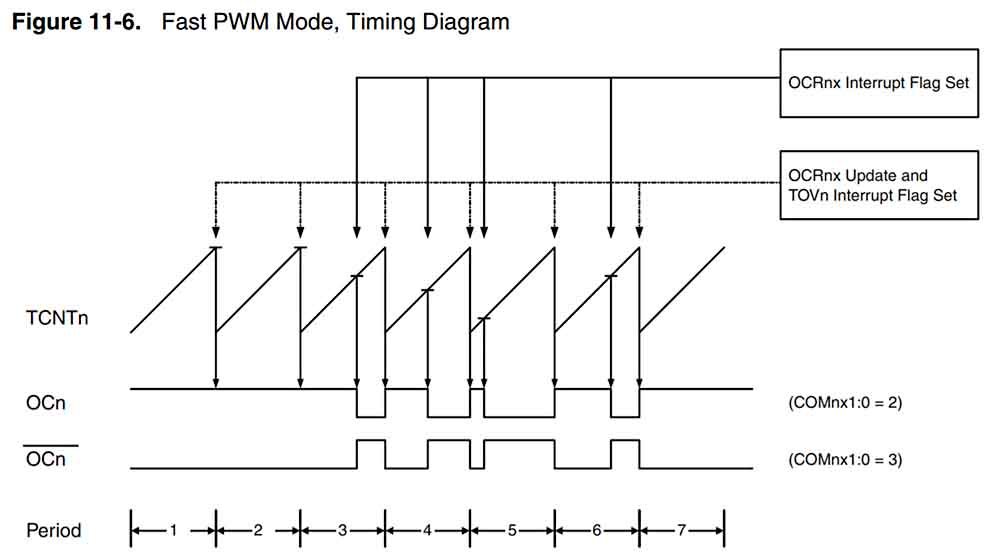Fast PWM mode flipping method.

### Code for the PWM output.¶

The coding was done in Arduino IDE. Settings and setup were the same as in the week 11 This week code continues of the learning in bit shifting and bit operators for register manipulation.

For this code, the Fast PWM register part of the datasheet was in heavy use.

From the datasheet, the register names and values for the Fast PWM were obtained and used in the code.

For example, the TCCR0A is 8- bit register that controls the mode of operation for the Timer/Counter0. Available modes are: non-PWM Mode, Fast PWM Mode, and Phase Correct PWM Mode. TCCR0A also controls the Waveform Generation mode that governs which of the available modes is activated.

To test the output of the week the potentiometer is set to a medium value to get a reading. For the current sense test, a resistor was soldered to the output to act as a dummy load. Same as the week 11.

The PWM signal was then measured from the board to test it is working.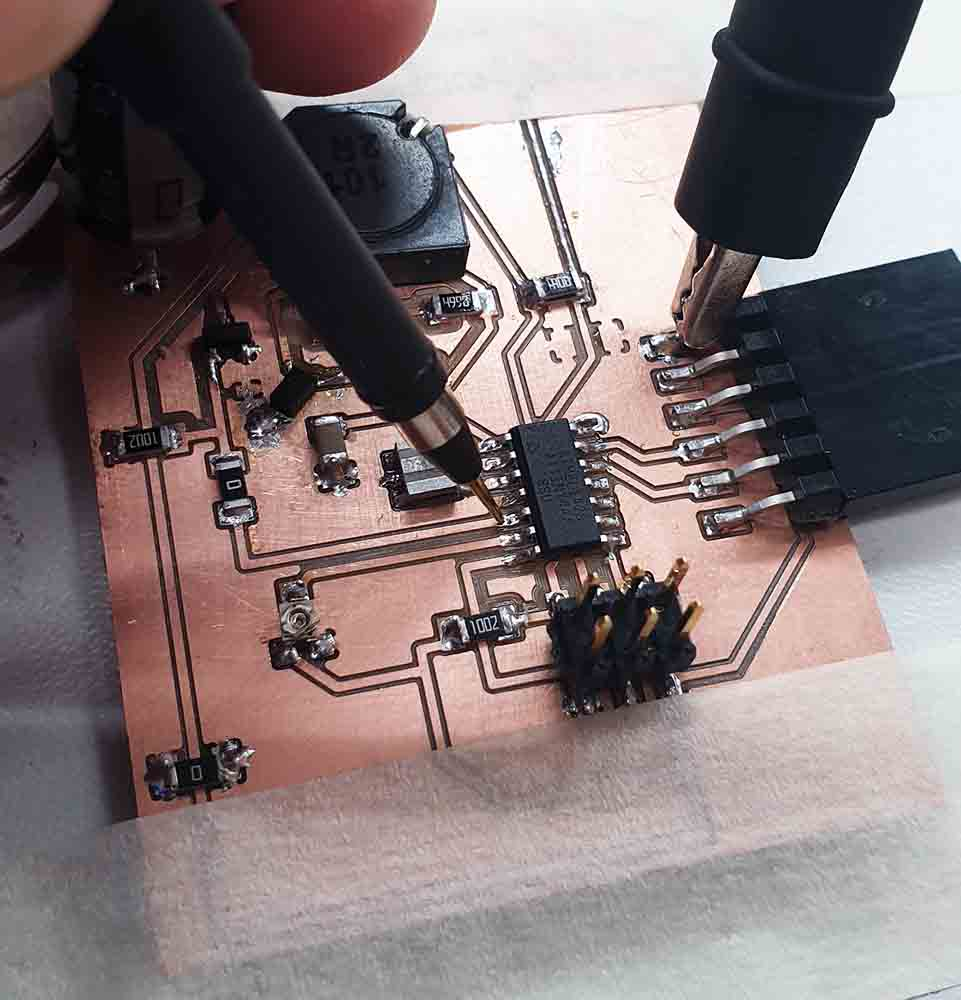PWM signal measure point.

The PWM output was first tested with regular AnalogWrite() function. This created 1.22Khz PWM signal.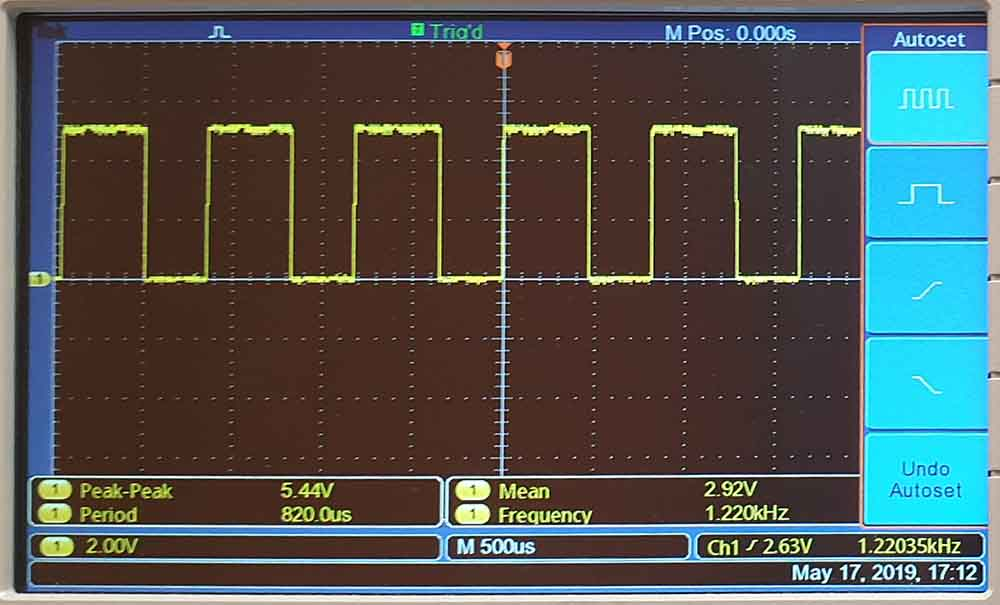First PWM signal out of the box.

The 1.22Khz is in the audible range. The coil whines very loudly and the frequency is horrifyingly irrating.

The prescaler for the PWM signal clock was then adjusted to 8. This created 20 000 000 Hz /(8*256)= 9 765 Hz signal for the PWM.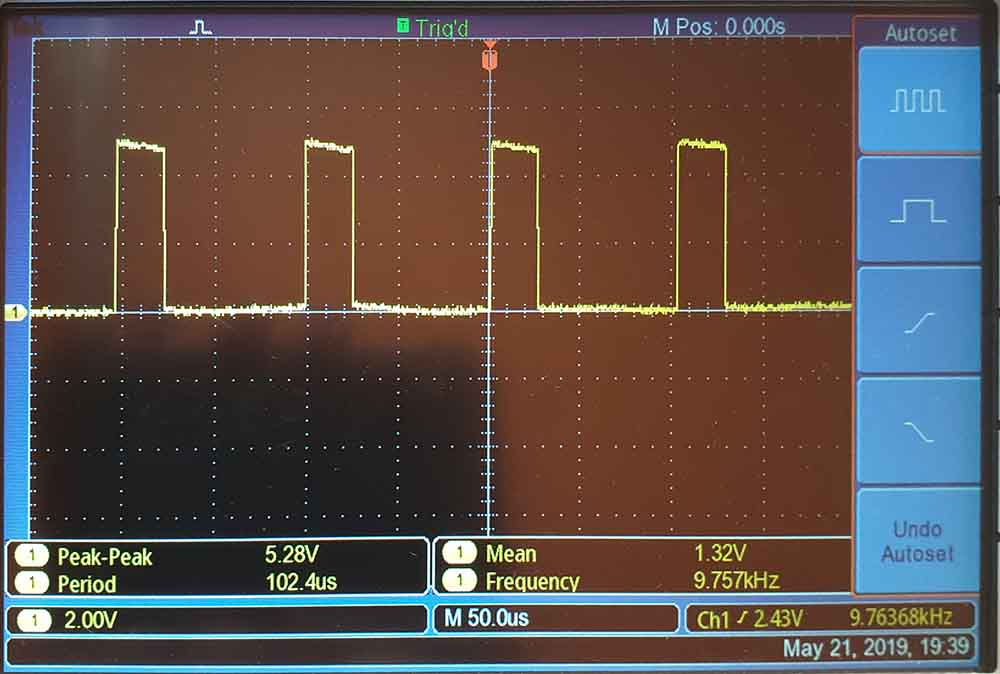Test PWM signal at 9.737kHz

This is still in audible range and makes horrible whining noise.

Then the prescaler was adjusted to 1 and a nice( 20 000 000 Hz /256=) 78 125 Hz PWM signal was optained.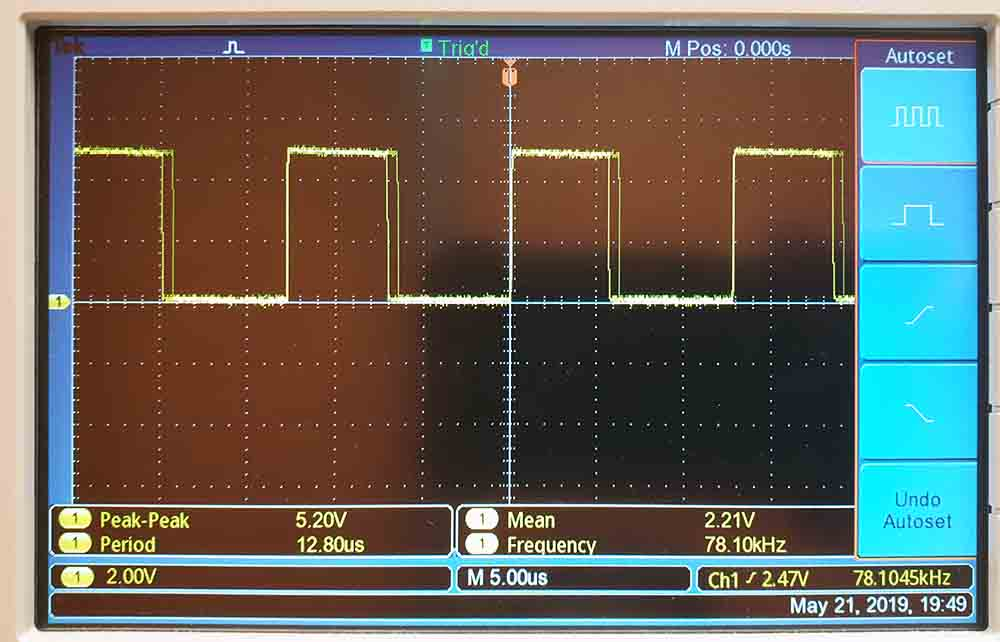Test PWM signal at 79.10kHz

Finally out of the audible range.Peace for human ears. Only bats will suffer.

```#ifndef F_CPU
#define F_CPU 20000000UL // 20 MHz clock speed
#endif

#include <avr/io.h>
#include <avr/interrupt.h>
#include <SoftwareSerial.h>         //serial ouput libary.

SoftwareSerial mySerial(0, 1); // RX, TX  // serial to output the read analog values.

volatile int VSense = 0;
volatile int Asense = 0;

void setup()
{
//here needs a set the PWM to low at first.

//THese are for the analog read pins:
ADCSRA    |=       (1 << ADPS2);                     // Set analog converter clock prescaler to 128 bit 3
ADCSRA    |=       (1 << ADPS1);                     // Set analog converter clock prescaler to 128 bit 2
ADCSRA    |=       (1 << ADPS0);                     // Set analog converter clock prescaler to 128bit 1

DIDR0     |=       (1<<ADC7D);                      // Disable digital input on port PA7
DIDR0     |=       (1<<ADC3D);                      // Disable digital input on port PA7
DIDR0     |=       (1<<ADC2D);                      // Disable digital input on port PA7

DDRA      &=      ~(1<<DDA7);                     //Make potentiometer pin an input.
DDRA      &=      ~(1<<DDA3);                     //Make Asense pin an input.
DDRA      &=      ~(1<<DDA2);                     //make Vsense pin an input.

ADMUX     &=      ~(1<< REFS0);                   // VCC used as analog reference, disconnected from PA0 (AREF)bit 0
ADMUX     &=      ~(1<< REFS1);                   // VCC used as analog reference, disconnected from PA0 (AREF)bit 1
ADMUX     |=       (1 << MUX2);                   // Start ADC7 for input (PA7), MUX bit 2
ADMUX     |=       (1 << MUX1);                   // start ADC7 for input (PA7), MUX bit 1
ADMUX     |=       (1 << MUX0);                   // start ADC7 for input (PA7), MUX bit 0 --> ADC7 (PA7) 111 Start from reading the potentiomater.

sei();                                              // Enable Global interrrupts

//these are for the PWM output,using the timer0:
DDRA      |=      (1<<DDB2);                           //setting the PWM pin to output.

TCCR0A    |=      (1<<WGM01);                    // set for Fast 8-bit PWM mode
TCCR0A    |=      (1<<WGM00);                    // set for Fast 8-bit PWM  mode
TCCR0A    |=      (1<<COM0A1);                   // Set OC0A on Compare MatchClear OC0A at BOTTOM (inverting mode). Driving N-channel mosfet.
TCCR0A    |=      (1<<COM0A0);                   // Set OC0A on Compare Match Clear OC0A at BOTTOM (inverting mode) Driving N-channel mosfet.
TCCR0B    |=      (1<<CS00);                    //  clk0/O/1 (No prescaling)try to go afap. this gives a freguency of 20 000 000hz/256= 78 125hz
TCCR0B    &=     ~(1<<CS01);                     //set CS01 to 0.

OCR0A = 255;                                   // start the PWM signal from 255 value (0-255 because of the 8-bit mode. 255 because of the inverted mode.)  P-MOSFET is off and no power is going through.

}

void loop()    // Don't do anything in loop. Everything happens only when interrupt comes from the ADC. Before there is nothing to do.
{

}         // end of main

{

switch (ADMUX)                                //Switch Case takes the measurement from the ADC and knows where from it came from and use the data accordingly.
{

break;

case 0x02:                                 // Case of register  ADMUX = 0b00000010 the case of reading the ADC2 output voltage

// At this point, we have enough information to make adjustments to the output PWM
while   (VSense  < potread){            // This checks if the output voltage is smaller than the set voltage. If the PWM is already small(and the MOSFET is fully open. Cannot do anymore- just write PWM as it is and continue to feed full voltage to the output.
if  (OCR0A == 0){
OCR0A = OCR0A ;
}

else {
OCR0A--;                   // if PWM is not at its highes, open the duty cycle more.
}

while     (VSense  > potread){          // This checks if the output voltage is higher than the set voltage. If the PWM is already big(and the MOSFET is fully closed. Cannot do anymore- just write PWM as it is and keep the MOSFET closed.
if  (OCR0A > 254){
OCR0A= OCR0A ;
}

else{
OCR0A++;                          // if the PWM is not set to low close the duty cycle more.
}
}
}

break;

case 0x03:                       // Case of register  ADMUX = 0b00000011,   ADC3 shunt resistor  voltage, Asense pin.

/*the voltage between before and after shunt resistor is Vdiff = Vsense - Asense. Max ampere we want to detect is 1Amp.
The shunt resistor will be 0.5 - ohm. From the ohm's law : V = IxR = 1amp*0.5ohm = 0.5v.
If the resolution of 8-bit ADC is 5/255= 0,01960V/ step then the difference betveen Vsense and V sense should be under 0.5V/ 0.01969V =  25.5 8 bit values. */
mySerial.print("Asense :");           // We have all the nessesary analog readings. push them out to serial
mySerial.print(Asense);
mySerial.print(" ");
mySerial.print("VSense :");
mySerial.print(VSense);
mySerial.print(" ");
if(VSense  - Asense > 30){        // step down the voltage if too much power is going trough.
OCR1A--;
}
ADMUX = 0x07;  // go back to the start

default:
//we newer should be here. something broke.
OCR1A = 0; //shutdown PWM
ADMUX = 0x07; // go to start.
break;

}

}
```

The output PWM will result a varying output voltage depending in the value of th potentiometer. With the code the PWM duty cycle is changed if the load in the output line is changed. Different resistors were tested on the device output and the output voltage was measured.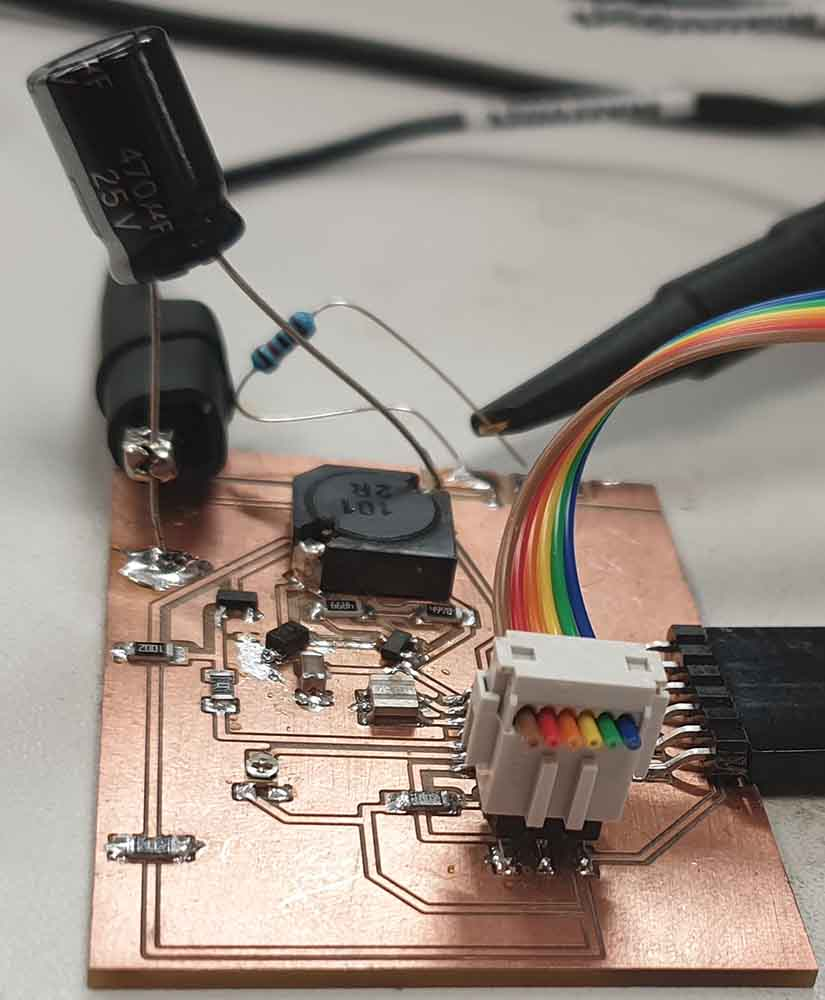Output voltage measure.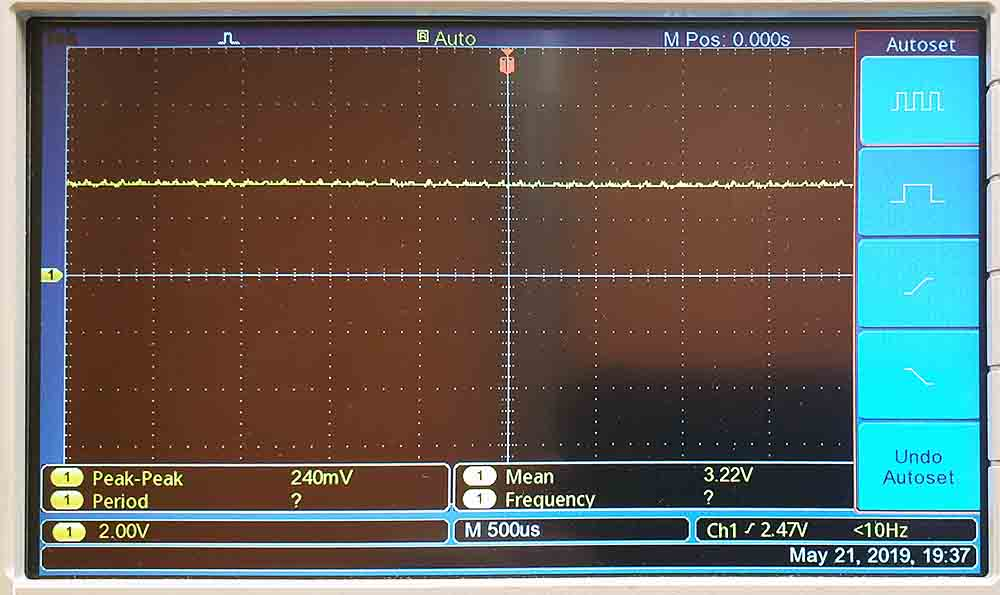Output voltage is stable.

All of my work files can be found at my gitlab repository

## Group work¶

Members: Lukasz, Gleb, Marjo, Jobin and I

Measure the power consumption of an output device.

#### 1. Green LED¶¶

For light emitting diodes, the results are visible with bare eye and connection is very simple. We found some random green LED on burned board. Our decision was to measure forward voltage to current characteristics. From this power would be easily calculated as Power = Voltage x Current.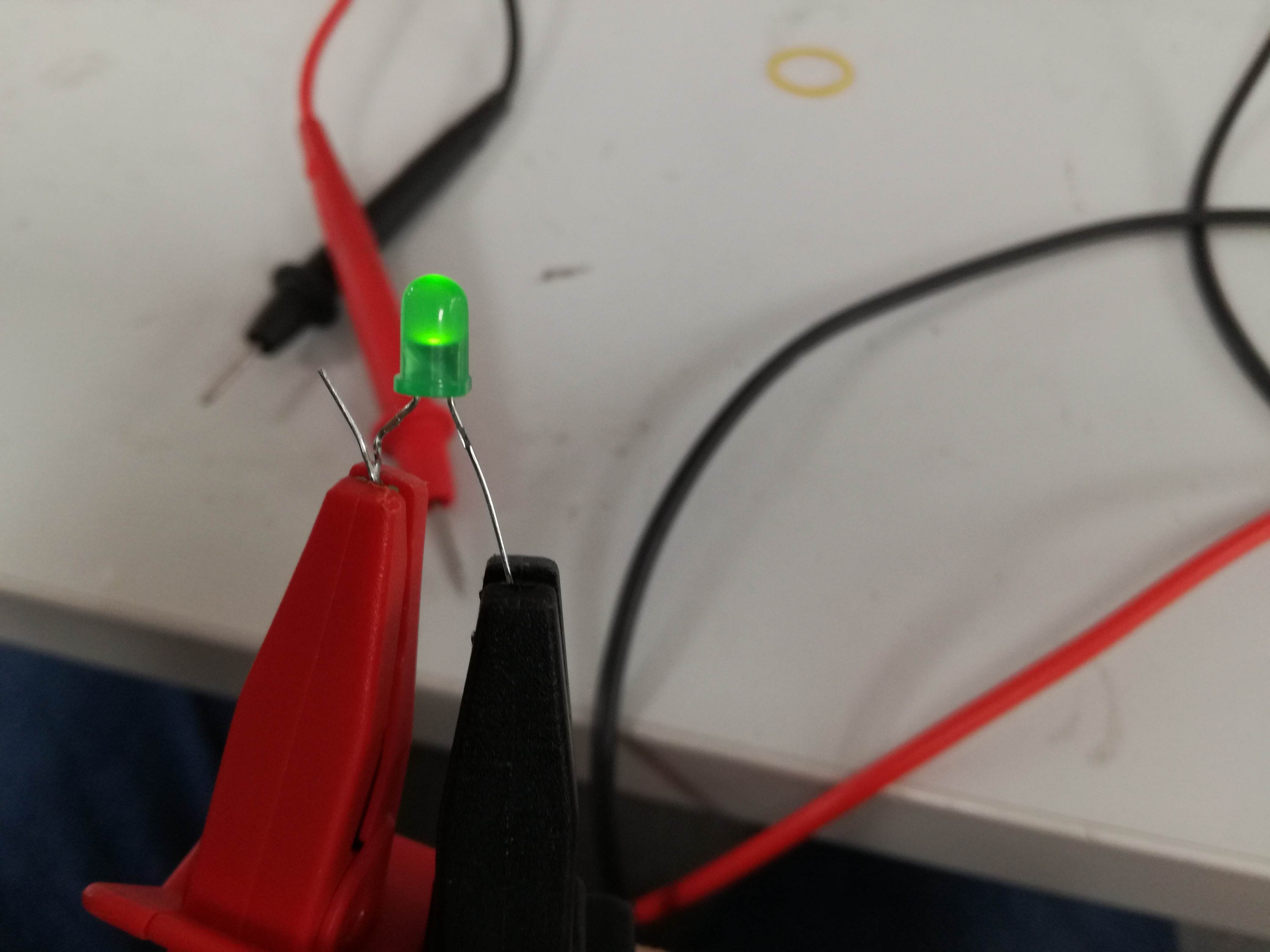The green led.

We connected the LED to the laboratory power supply. It is a smart construction as it has both current and voltage limited output. It would be really difficult to burn the LED but we managed to do that.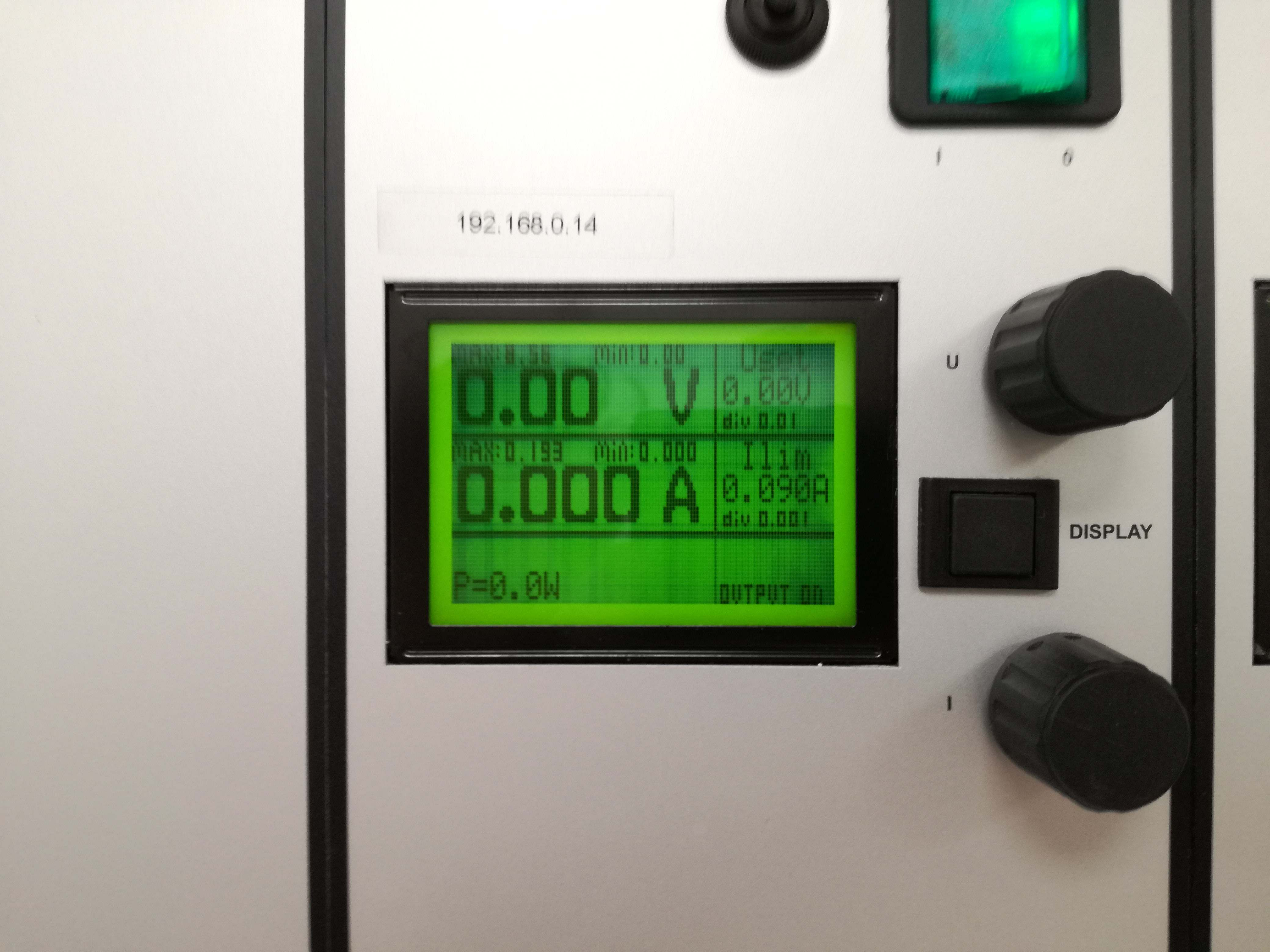Bench power supply.

We were feeding LED with voltage, where current was limited every time. We obtained full characteristics from barely glowing to final breath of our LED. Results are shown below.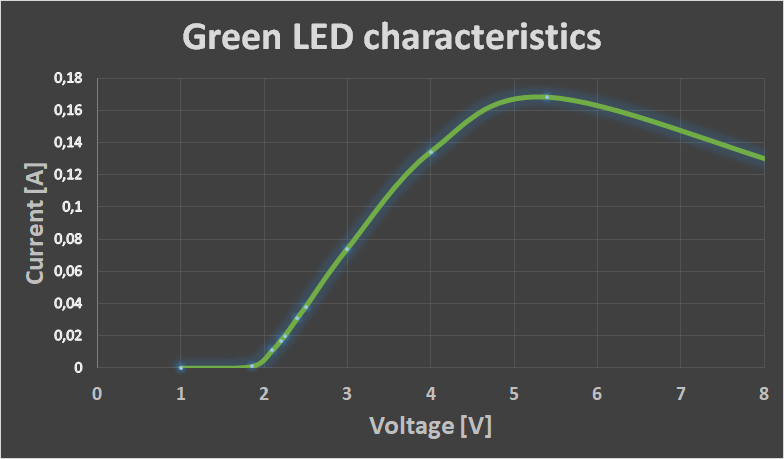Graph for the LED.

The measured values in table: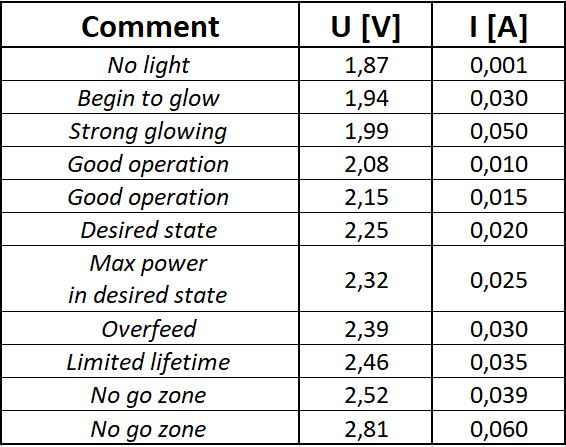Table of values LED.

#### 2. Motherboard Fan¶

Our next choice was a cooling fan. This was doing its best to cool down some electrical circuit. Nominal values were 12 Volts at 0.9 Ampere. Once again we used our laboratory power supply. This time we limited current to the maximum value of 0.9 Amp and played with voltage. We obtained both Current vs Voltage and Power vs Voltage characteristics.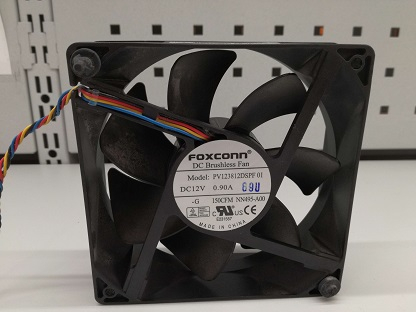120x25mm brushless fan.

We wrote down our observations during measuring process. We observed that at some very low voltage it was necessary to manually start the fan and it keeps spinning (slow and with issues). Before it starts it is trying to compensate lack of voltage with current. It is unfortunately not enough. Table with our results and observations below: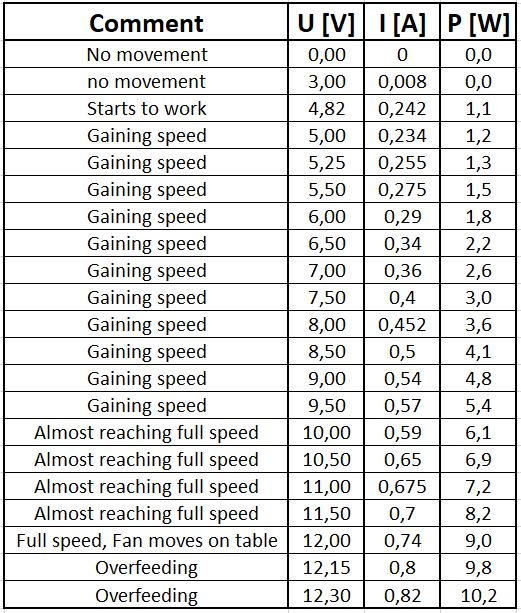Table of measured values for the Fan.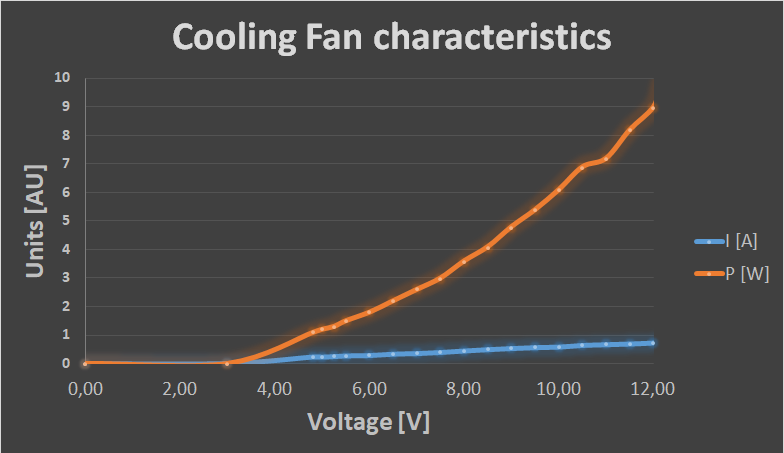Graph from the tables values,